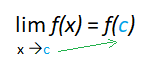# Direct Substitution: Find Limits in Easy Steps

## Finding Limits with Direct SubstitutionDirect substitution is just what the name implies: you directly substitute a given value into a limit.

Probably the most intuitive way to find a limit is to look at a limit graphically (on a graphing calculator) or numerically (through a table). However, in some cases it’s actually easier—and faster—to find a limit with direct substitution. If you’ve ever put a specific value into an equation in algebra (like putting x = 2 into the function y = x + 10), then you have already performed direct substitution. It’s really that easy!

## When to Use Direct Substitution

Direct substitution works well when you have a simple functions with additions, subtractions, divisions, multiplications, powers and roots.

For example, this technique can work with constant functions, like:

• f(x) = 10,
• f(x) = 6,
• f(x) = 99.99.

You can also use it to find limits for linear functions, which produce straight lines in a graph; they have “x” as the input variable and the “x” has an exponent of one. Examples of linear functions are:

• f(x) = x,
• f(x) = 2x – 2,
• f(x) = x + 1.

When NOT to use direct substitution: If you plug in x-values (using the steps below) and get an indeterminate limit (either 0/0 or ∞/∞), you can’t use this technique. Use the dividing out technique for limits instead.

## Example problem 1:

Find the limit of f(x) = 9x – 2 at x = 6.

Step 1: Make sure the function as a simple function (one of the types shown in the above image). This particular function is made up of sums, so we can use the rule.

Step 2: Substitute the “x” value (the point at which you want to find the limit) into the function. In this example, you’re looking for the limit at x = 6, so:

f(x) = 9x – 2
f(x) = 9(6) – 2
f(x) = 54 – 2
f(x) = 52

The limit of f(x) = 9x – 2 as x approaches 6 is 52.

A quick look at a graph of f(x) = 9x – 2 shows this is the correct answer:## Example 2

Find the limit of f(x) = √(x) at x = 4

Step 1: Make sure the function as a simple function. This is a radical function, so we can use the rule.

Step 2: Substitute the “x” value into the function. In this example, you’re looking for the limit at x = 4, so:
√(4) = 2

The limit of f(x) = √(x) as x approaches 4 is 2.

## Example 3

This next example may look a little more complicated, but it isn’t: you’re still just “filling in the blanks.”

Find the limit:Step 1: Make sure the function as a simple function. This is a radical function, so we can use the rule.

Step 2: Substitute the “x” value into the function. In this example, you’re looking for the limit at x = 6, so:The limit as x approaches 6 is 4.